Next: Dan Kucervosky - An Up: Operator Algebras / Algèbres Previous: George Elliott - An

## Thierry Giordano - Ergodic theory and dimension G-spaces

 THIERRY GIORDANO, University of Ottawa, Ottawa, Ontario  K1N 6N5, Canada Ergodic theory and dimension G-spaces

Let G be a discrete group. The real group algebra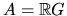has a natural order structure given by the positive cone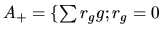a.e. and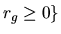and is endowed with an order-preserving action of G (by right multiplication). If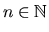, then An is a partially ordered vector space with the direct sum ordering and a G-space with the above G-action.

Definition. A G-dimension space H is a partially ordered vector space with an action of G (as a group of order automorphisms) that can be obtained as a direct limit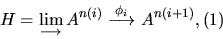where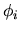is a positive A-module map (for the natural ordering on A).

Corresponding to the inductive limit in (1) is a matrix-valued random walk on G. The harmonic functions associated to this random walk are in a natural bijection with the states on H. A state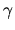corresponding to a bounded harmonic function is called bounded. It induces a pseudo-norm on H and allows us to associate to H the real L1-space L1(X), the completion'' of H (as defined by Goodearl and Handelman). If for all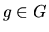,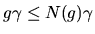(N(g)depending on g), then G acts on L1(X).

D.E. Handelman and I have defined the notion of ergodicity and different generalizations of approximate transitivity for the action of G on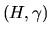which extends to L1(X) and its dual.Next: Dan Kucervosky - An Up: Operator Algebras / Algèbres Previous: George Elliott - An